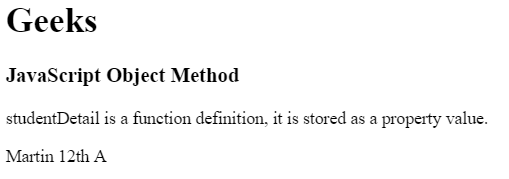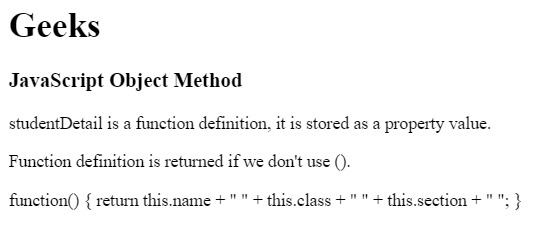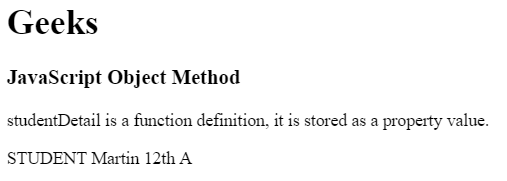# JavaScript | Object Methods

Object Methods in JavaScript can be accessed by using functions. Functions in JavaScript are stored as property values. The objects can also be called without using bracket ().

• In a method, ‘this’ refers to the owner object.
• Additional information can also be added along with the object method.

Syntax:

`objectName.methodName()`

Properties: A function may be divided into different property values, which are then combined and returned together.
For Ex: Student function contains the properties:

• name
• class
• section

Return Value: It returns methods/functions stored as object properties.

Example 1: This example use function definition as property value.

 ` ` `<``html``> ` ` `  `<``head``> ` `    ``<``title``> ` `        ``JavaScript Object Methods ` `    `` ` ` ` ` `  `<``body``> ` `    ``<``h1``>Geeks ` `     `  `    ``<``h3``>JavaScript Object Method ` `     `  `    ``<``p``> ` `        ``studentDetail is a function definition, ` `        ``it is stored as a property value. ` `    `` ` `     `  `    ``<``p` `id``=``"gfg"``> ` `     `  `    ``<``script``> ` `     `  `        ``// Object creation ` `        ``var student = { ` `            ``name: "Martin", ` `            ``class : "12th", ` `            ``section : "A", ` `             `  `            ``studentDetails : function() { ` `                ``return this.name + " " + this.class  ` `                    ``+ " " + this.section + " "; ` `            ``} ` `        ``}; ` `         `  `        ``// Display object data ` `        ``document.getElementById("gfg").innerHTML  ` `                ``= student.studentDetails(); ` `    `` ` ` ` ` `  `                                 `

Output:Example 2: This example use storing property values and accessing without bracket ().

 ` ` `<``html``> ` ` `  `<``head``> ` `    ``<``title``> ` `        ``JavaScript Object Methods ` `    `` ` ` ` ` `  `<``body``> ` `    ``<``h1``>Geeks ` `     `  `    ``<``h3``>JavaScript Object Method ` `     `  `    ``<``p``> ` `        ``studentDetail is a function definition, ` `        ``it is stored as a property value. ` `    `` ` `     `  `    ``<``p``> ` `        ``Function definition is returned ` `        ``if we don't use (). ` `    `` ` `    ``<``p` `id``=``"gfg"``> ` `     `  `    ``<``script``> ` `     `  `        ``// Object creation ` `        ``var student = { ` `            ``name: "Martin", ` `            ``class : "12th", ` `            ``section : "A", ` `             `  `            ``studentDetails : function() { ` `                ``return this.name + " " + this.class ` `                    ``+ " " + this.section + " "; ` `            ``} ` `        ``}; ` `         `  `        ``// Display object data ` `        ``document.getElementById("gfg").innerHTML  ` `                ``= student.studentDetails; ` `    `` ` ` ` ` `  `                                 `

Output:Example 3: Using function definition as property value and accessing with additional details.

 ` ` `<``html``> ` ` `  `<``head``> ` `    ``<``title``> ` `        ``JavaScript Object Methods ` `    `` ` ` ` ` `  `<``body``> ` `    ``<``h1``>Geeks ` `     `  `    ``<``h3``>JavaScript Object Method ` `     `  `    ``<``p``> ` `        ``studentDetail is a function definition, ` `        ``it is stored as a property value. ` `    `` ` `     `  `    ``<``p` `id``=``"gfg"``> ` `     `  `    ``<``script``> ` `     `  `        ``// Object creation ` `        ``var student = { ` `        ``name: "Martin", ` `        ``class : "12th", ` `        ``section : "A", ` `         `  `        ``studentDetails : function() { ` `            ``return this.name + " " + this.class  ` `                ``+ " " + this.section + " "; ` `        ``} ` `    ``}; ` `     `  `    ``// Display object data ` `    ``document.getElementById("gfg").innerHTML ` `        ``= "STUDENT " + student.studentDetails(); ` `    `` ` ` ` ` `  `                                     `

Output:My Personal Notes arrow_drop_upCheck out this Author's contributed articles.

If you like GeeksforGeeks and would like to contribute, you can also write an article using contribute.geeksforgeeks.org or mail your article to contribute@geeksforgeeks.org. See your article appearing on the GeeksforGeeks main page and help other Geeks.

Please Improve this article if you find anything incorrect by clicking on the "Improve Article" button below.

Article Tags :

Be the First to upvote.

Please write to us at contribute@geeksforgeeks.org to report any issue with the above content.